# Find the surface area of the water in the given prism

• chwala

#### chwala

Gold Member
Homework Statement
See attached ( past paper question)
Relevant Equations
Knowledge of prisms
My query in only on the highlighted part...c.ii.

Find the question below;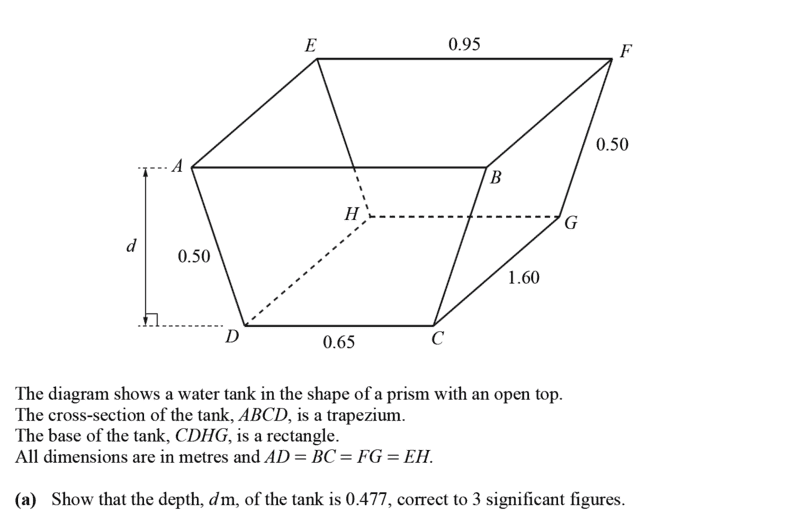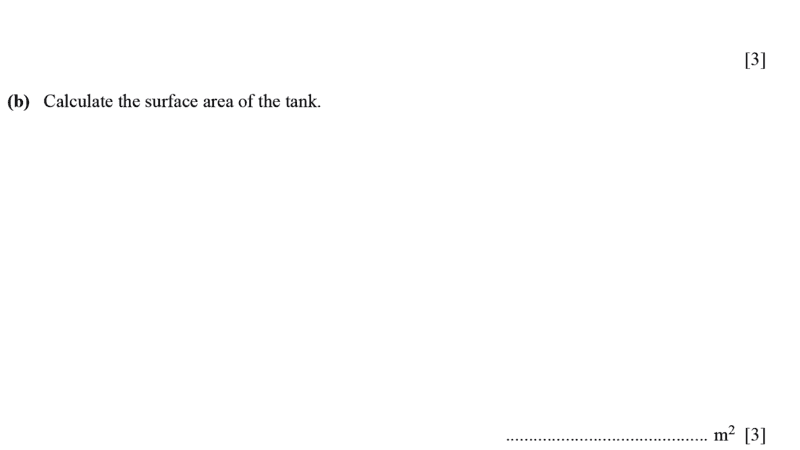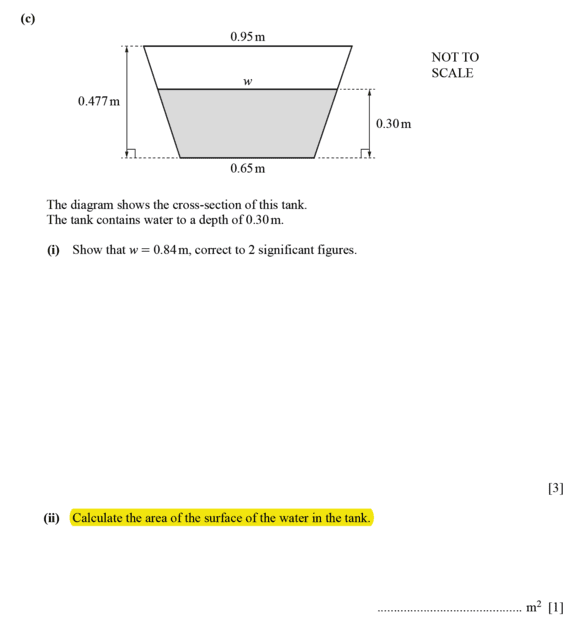Find the markscheme here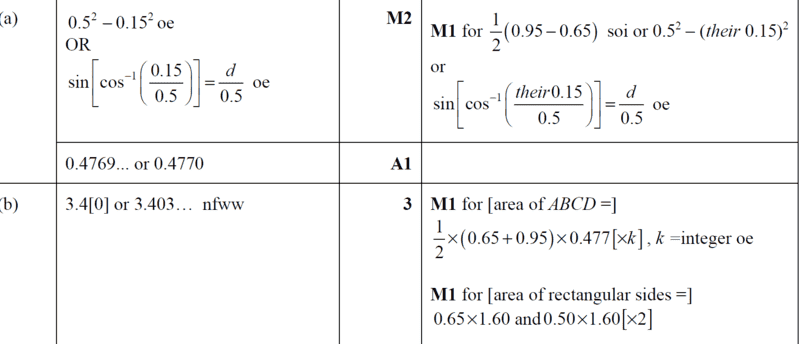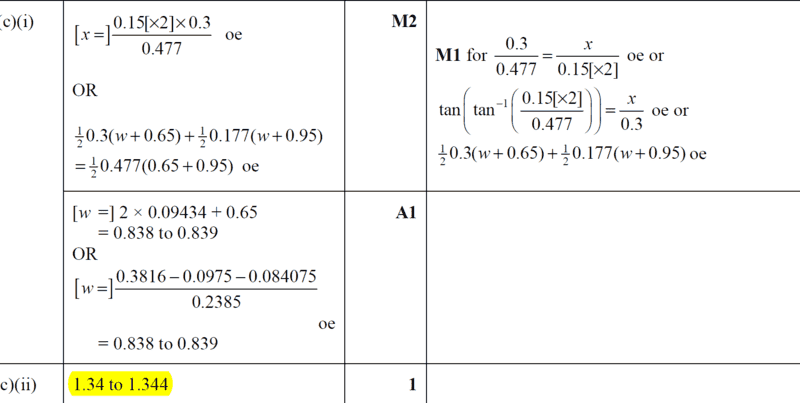part c(ii) does not seem correct as i have;
##A_1=0.5 ×(0.65+0.84)0.3 ×2=0.447m^2##
##A_2 = 0.65 ×1.6=1.04m^2##
##A_3 = (0.3146 × 1.6)2=1.00672m^2##
Total surface area = ##A_1+A_2+A_3=0.447+1.04+1.00672=2.494m^2##...

For part c.(i), i was able to use similarity (i used a right angle triangle from the cross-section) to show that,
i.e ##\dfrac {0.477}{0.15}##=##\dfrac {0.3}{x}##
##x=0.094## therefore, ##w = 0.65+2(0.094)=0.8386≈0.84## to 3 2 significant figures.

Last edited:
•Delta2

aaargh! its the english man, i figured it out, ##0.84 × 1.6! ## Area of surface and not Surface area! phew##x=0.094## therefore, ##w = 0.65+2(0.094)=0.8386≈0.84## to 3 significant figures.
0.8386 to 3 significant figures amounts to 0.839(i.e. a leading zero does not count as a significant figure)

##\ ##

0.8386 to 3 significant figures amounts to 0.839(i.e. a leading zero does not count as a significant figure)

##\ ##...to two significant figures...yeah, i know that. cheers bvu...